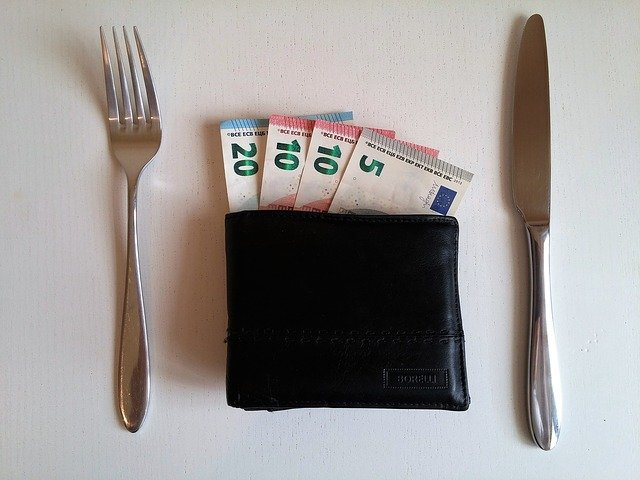30 February 2021

# What is Houdini Predictions Football Betting Calculator?There are three most common odds formats, and here, we're going to take a look at each of them.

American odds (also known as Moneyline) are based on banking \$100 for a particular bet. When it comes to this type of odds, the favorites will have a minus (-) sign in front, which reflects the bet you need to place to earn \$100. In the meantime, the odds for the betting outsiders will have a plus (+) sign, representing your potential win for a \$100 wager.

Few bettors use fractional odds for betting sports, as the conversions to understand revenue are complex. To calculate winnings on fractional odds, you have to multiply your bet by the top number, and then divide the result by the bottom. Therefore, a \$100 wager at 5/2 odds is (100 * 5) / 2, which equals \$250.

Using decimal odds makes the bettors' life quite simple because it's pretty straightforward to calculate the result by simply multiplying them with the bet. So, decimal odds represent the amount won by a gambler for every \$1 wagered. Unlike American and fractional odds, the number shows the total income and not only the profit. Let's say you risk \$1 at odds of 1.8 - you're profiting 0.80 cents, plus your initial \$1 back.

## Why you should use our betting odds calculator?

No matter where you come from or what odds format you prefer, we at Houdini Predictions are here to make your betting experience as smooth as you can imagine. In order to give you the chance to pick your own way of calculating your return, we introduce you to the online betting calculator. Indeed, the betting odds calculator enables you to determine the payout by calculating your stake and odds in any of the abovementioned formats.

Our football betting calculator does the hard work for you, allowing you to find out the result in any given odds format in a matter of seconds. So, you don’t need to have any mathematical knowledge to be able to exploit our best football betting tips for tonight, as you can just let our betting odds calculator do the maths for you. It's only one of many sophisticated tools Houdini Predictions offer to save you time and needless efforts.

How our online betting calculator works?

Let’s see how you can convert fractional odds into decimal. Since these two formats are the most common ones, we are going to focus on them here.

To do the conversion, you have to break the fraction into a decimal format and add 1. That practically means you need to divide the numerator (the number left of the divider) by the denominator (the number right of the divider).

Here's an example - if you want to convert fractional odds of 1/1 into a decimal format, you have to do the following calculation.

1/1 = 1

1+1 = 2

Simple as that!

To convert decimal odds to a fractional format, you just have to reverse the process. In this case, the decimal number serves as the numerator and 1 as the denominator. Then you have to multiply the numbers on the left and right by 10 until there are no decimal spots left.

If you want to convert decimal odds of 2.00 into fractional odds, do the following.

2-1 = 1

(1.00/1) * 10 = 1/1

However, since this may seem too complicated, you can just use our betting odds calculator to accelerate the process and get the results in seconds with a single mouse click